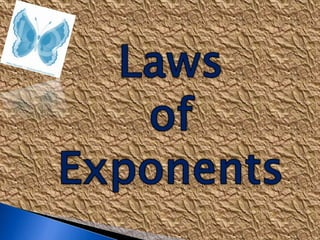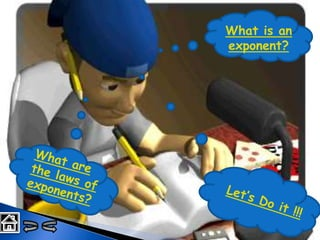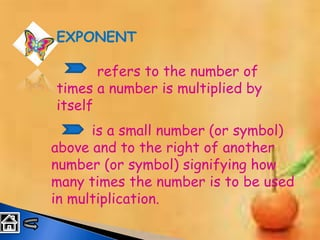Successfully reported this slideshow.

# Exponents

4

Share×
1 of 42
1 of 42

# Exponents

4

Share

Definition and laws of exponents

Definition and laws of exponents

## More Related Content

### Related Books

Free with a 30 day trial from Scribd

See all

### Related Audiobooks

Free with a 30 day trial from Scribd

See all

### Exponents

1. 1. What is an exponent?
2. 2. EXPONENT refers to the number of times a number is multiplied by itself is a small number (or symbol) above and to the right of another number (or symbol) signifying how many times the number is to be used in multiplication.
3. 3. LAWS OF EXPONENTS
4. 4. LAWS OF EXPONENTS LAW # 1 LAW # 4 LAW # 3 LAW # 2 LAW # 5
5. 5. LAWS OF EXPONENTS xmxn = xm+n LAW # 1 Product of a Power
6. 6. LAWS OF EXPONENTS LAW # 2 Power of Powers (xm)n = xmn
7. 7. LAWS OF EXPONENTS LAW # 3 Quotient of a Power xm/xn = xm-n
8. 8. LAWS OF EXPONENTS LAW # 4 Zero Exponent x0 = 1
9. 9. LAWS OF EXPONENTS LAW # 5 Negative Exponent x-1 = 1/x
10. 10. LET’S DO IT !!! Simplify each of the following using the laws of exponents. Click the letter of your answer. (3x)( 4x²) 12x² 12x³ 1
11. 11. LET’S DO IT !!! Simplify each of the following using the laws of exponents. Click the letter of your answer. (3a³b)² 9a⁶b² 6a⁶b² 2
12. 12. LET’S DO IT !!! Simplify each of the following using the laws of exponents. Click the box of your answer. (2a³b²)(3a³b) 9a⁶b² 6a⁶b³ 3
13. 13. LET’S DO IT !!! Simplify each of the following using the laws of exponents. Click the letter of your answer. (2a²bc)³ 8a⁶b³c³ 6a⁶b³c 4
14. 14. LET’S DO IT !!! Simplify each of the following using the laws of exponents. Click the letter of your answer. (2m²n)(4mn) (m³n²) 8m⁶n² 8m⁶n⁴ 5
15. 15. How ‘bout this ? Simplify each of the following using the laws of exponents. Click the letter of your answer. 1 2 3 a. 4a³ b. 4a a. 2x²y b. -2x²y a. 1 b. 0
16. 16. Simplify each of the following using the laws of exponents. Click the letter of your answer. Let’s explore!!! 1
17. 17. Simplify each of the following using the laws of exponents. Click the letter of your answer. Let’s explore!!! 2
18. 18. Simplify each of the following using the laws of exponents. Click the letter of your answer. Let’s explore!!! 3
19. 19. THE END !!!
20. 20. THANK YOU !!! Prepared by: Maria Lourdes A. Manandig Jaime J. Vistan High School Tabang, Plaridel, Bulacan